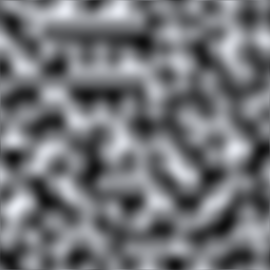Version: 5.6

# Mathf.PerlinNoise

マニュアルに切り替える
public static float PerlinNoise (float x, float y);

## パラメーター

 x サンプル点の X 座標 y サンプル点の Y 座標

## 戻り値

float 0.0 と 1.0 の間の値

## 説明

2D のパーリンノイズを生成します

Perlin noise is a pseudo-random pattern of float values generated across a 2D plane (although the technique does generalise to three or more dimensions, this is not implemented in Unity). The noise does not contain a completely random value at each point but rather consists of "waves" whose values gradually increase and decrease across the pattern. The noise can be used as the basis for texture effects but also for animation, generating terrain heightmaps and many other things.Perlin noise sampled in the range 0..10 (the greyscale values represent values from 0..1)

Any point in the plane can be sampled by passing the appropriate X and Y coordinates. The same coordinates will always return the same sample value but the plane is essentially infinite so it is easy to avoid repetition by choosing a random area to sample from.

```using UnityEngine;
using System.Collections;public class ExampleClass : MonoBehaviour {
public int pixWidth;
public int pixHeight;
public float xOrg;
public float yOrg;
public float scale = 1.0F;
private Texture2D noiseTex;
private Color[] pix;
private Renderer rend;
void Start() {
rend = GetComponent<Renderer>();
noiseTex = new Texture2D(pixWidth, pixHeight);
pix = new Color[noiseTex.width * noiseTex.height];
rend.material.mainTexture = noiseTex;
}
void CalcNoise() {
float y = 0.0F;
while (y < noiseTex.height) {
float x = 0.0F;
while (x < noiseTex.width) {
float xCoord = xOrg + x / noiseTex.width * scale;
float yCoord = yOrg + y / noiseTex.height * scale;
float sample = Mathf.PerlinNoise(xCoord, yCoord);
pix[y * noiseTex.width + x] = new Color(sample, sample, sample);
x++;
}
y++;
}
noiseTex.SetPixels(pix);
noiseTex.Apply();
}
void Update() {
CalcNoise();
}
}
```

Although the noise plane is two-dimensional, it is easy to use just a single one-dimensional line through the pattern, say for animation effects.

```using UnityEngine;
using System.Collections;public class ExampleClass : MonoBehaviour {
public float heightScale = 1.0F;
public float xScale = 1.0F;
void Update() {
float height = heightScale * Mathf.PerlinNoise(Time.time * xScale, 0.0F);
Vector3 pos = transform.position;
pos.y = height;
transform.position = pos;
}
}
```

Note: It is possible for the return value to slightly exceed 1.0f. You may need to clamp the return value if the 0.0 to 1.0 range is important to you.